# 同态加密soolaugust﻿

﻿

《数据价值-联邦学习篇》：初识 中我们在说联邦学习时提到过联邦学习使用同态加密技术来保证数据的安全。并简单介绍了一下同态加密，今天我们来详细说一下同态加密是什么，并以一个同态加密方案来说明。

﻿﻿

## 什么是同态加密

﻿

﻿

﻿

﻿

﻿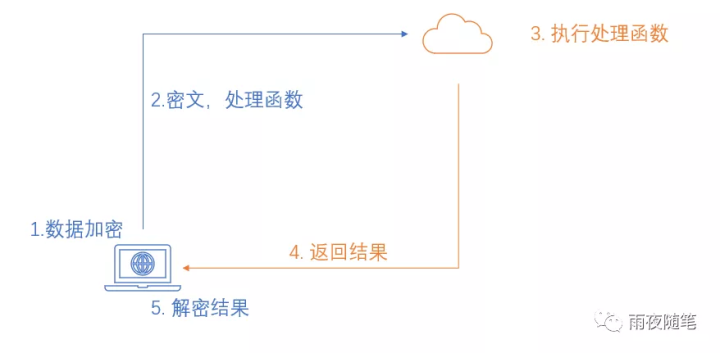﻿

﻿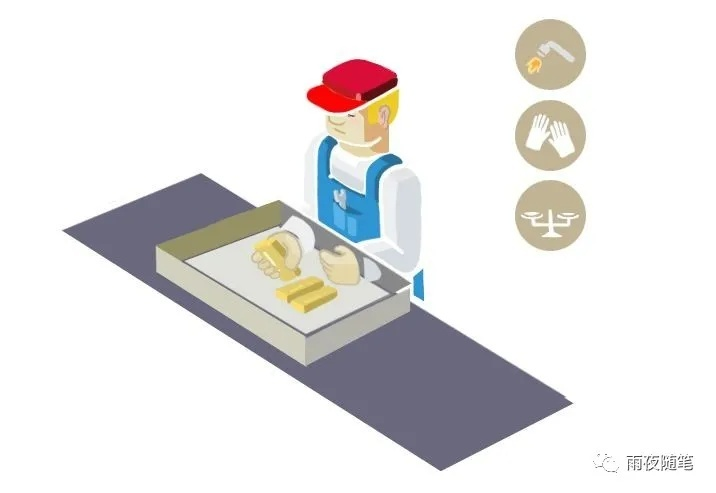﻿

﻿

﻿

,然后云端返回这个结果，我们再进行解密，就得到了我们想要的结果 。

﻿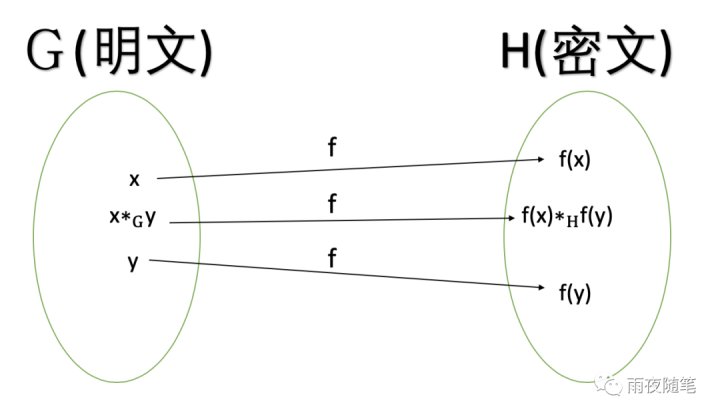﻿

﻿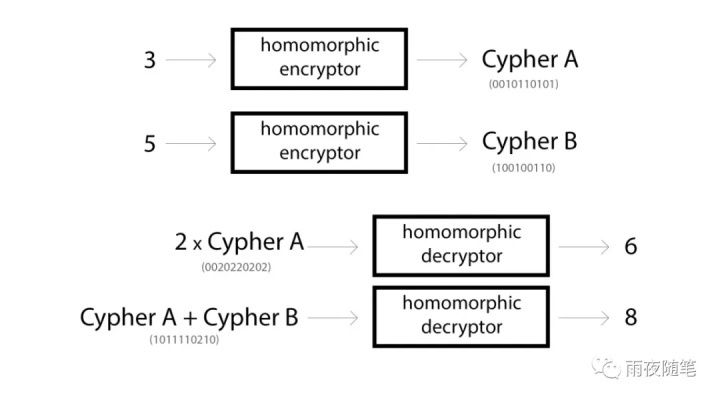﻿

## semantic security

﻿

﻿

﻿

• SWHE：这种类型的同态加密只支持特定的函数，比如加法，或者乘法，或者加乘法等。

• FHE：这种类型的同态加密支持任意的函数，只要这种函数可以通过算法描述，并用计算机能够实现。

﻿

﻿

﻿

• KeyGen：即密钥生成函数。主要是生成数据加解密所需的密钥。

• Encrypt：加密函数，主要是使用密钥对数据进行加密。

• Decrypt：解密函数，主要是使用密钥对数据进行解密。

• Evaluate：这个主要是对加密后的数据进行计算，一般用于用户编写自己的处理方法。

﻿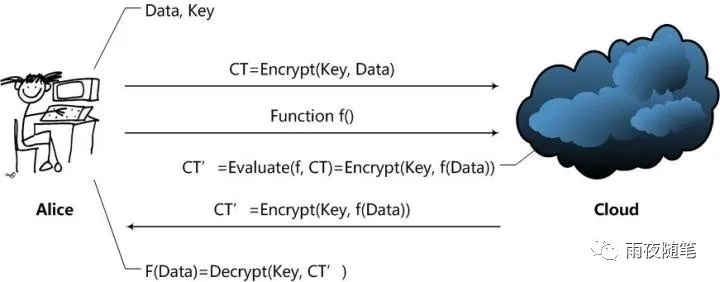﻿

﻿

﻿

﻿

## RSA算法

﻿

﻿

1. 随机选择两个不同的素数p,q

2. 取p,q的乘积n，即

3. 根据欧拉函数，当p,q为不同的素数时，有

4. 然后随机选择一个整数e，满足两个条件：与e互质，并且1<e<

5. 计算e对于 的模反元素d，也就是满足ed-1=k ,一般写作

6. 最终(e, n)为公钥，(d,n)为私钥

7. 加密数据m的方式是：

8. 解密的方式是：

﻿

﻿

﻿

﻿

1. 我们现在有公钥(e, n)，如果我们能够计算出d，就能拿到私钥。

2. 因为 ，我们已经知道了e，所以只要知道就可以了。而，所以我们需要知道p,q的值。而，所以我们要做的其实是分解n。但是n一般都很大，大数分解就是RSA所依赖的数学难题。目前可以破解的最大整数是768位（二进制）整数。所以大于768位的RSA算法目前可以认为是安全的。

## Paillier cryptosystem

﻿

﻿

• 首先是密钥生成函数KeyGen

﻿

1. 选取两个不同的素数p,q

2. 取两个数的乘积

3. 据欧拉函数，当p,q为不同的素数时，有,这里记为

4. 然后随机选择一个整数g，, 并且和n互质

5. 然后取 , 这里的

6. 然后取(n,g )为公钥，（λ，μ）为私钥

﻿

// KeyGen 秘钥生成函数 random是随机生成源， 用于生成随机数。bits是生成随机数的大小func KeyGen(random io.Reader, bits int) (*PubKey, *PrivKey, error) {  // 随机素数 p  p, err := rand.Prime(random, bits/2)  if err != nil {    return nil, nil, err  }  // 随机素数 q  q, err := rand.Prime(random, bits/2)  if err != nil {    return nil, nil, err  }  // N = p*q  n := new(big.Int).Mul(p, q)  // 这里为了计算方便，保存 n * n  nSquared := new(big.Int).Mul(n, n)  // 随机整数g，这里问了方便为 n+1  g := new(big.Int).Add(n, one)  // p-1  pMin := new(big.Int).Sub(p, one)  // q-1  qMin := new(big.Int).Sub(q, one)  // (p-1)*(q-1)  l := new(big.Int).Mul(pMin, qMin)  // l^-1 mod n  u := new(big.Int).ModInverse(l, n)  // 公钥保存n, g, n*n  pub := &PubKey{Len: bits, N: n, Nsq: nSquared, G: g}  // 私钥保存l, u, n, g, n*n  return pub, &PrivKey{PubKey: *pub, Len: bits, L: l, U: u}, nil}

﻿

• 然后是加密函数Encrypt，待加密数据是m, r为选择的随机数，0 < r < n，并且和n互质

﻿

// Encrypt函数， 加密数据// c = g^m * r^n mod n^2// 0 <= r <= n// message是待加密的数据func Encrypt(pubkey *PubKey, message []byte) ([]byte, error) {  // r是随机素数  r, err := rand.Prime(rand.Reader, pubkey.Len)  if err != nil {    return nil, err  }  // 将信息转换成整数，方便计算  m := new(big.Int).SetBytes(message)  if pubkey.N.Cmp(m) < 1 {    return nil, errors.New("ras算法要求对加密的信息有要求，要求不能大于大于n，否则会发生mod n会发生回绕，是的解密发生错误")  }  //g^m  gm := new(big.Int).Exp(pubkey.G, m, pubkey.Nsq)  //r^n  rn := new(big.Int).Exp(r, pubkey.N, pubkey.Nsq)  // prod = g^m * r^n  prod := new(big.Int).Mul(gm, rn)  c := new(big.Int).Mod(prod, pubkey.Nsq)  return c.Bytes(), nil}

﻿

• 解密函数Decrypt

﻿

// Decrypt函数， 解密数据// m = L(c^lamda mod n^2).mu mod nfunc Decrypt(privkey *PrivKey, cipher []byte) ([]byte, error) {  // 将带解密的密文转换成整数，进行计算  c := new(big.Int).SetBytes(cipher)  // 如果 n*n < c  if privkey.Nsq.Cmp(c) < 1 {    return nil, errors.New("ras算法要求对加密的信息有要求，要求不能大于大于n，则密文不能大于n*n")  }  //c^lamda mod n^2  a := new(big.Int).Exp(c, privkey.L, privkey.Nsq)  //L(x) = x-1 / n  l := new(big.Int).Div(new(big.Int).Sub(a, one), privkey.N)  //computing m  m := new(big.Int).Mod(new(big.Int).Mul(l, privkey.U), privkey.N)  return m.Bytes(), nil}

﻿

• 计算函数Evaluate

﻿

﻿

// 加法1func Add(pubkey *PubKey, c1, c2 []byte) []byte {  a := new(big.Int).SetBytes(c1)  b := new(big.Int).SetBytes(c2)  // a * b mod n^2  res := new(big.Int).Mod(new(big.Int).Mul(a, b), pubkey.Nsq)  return res.Bytes()}

﻿

﻿

//加法2func AddConstant(pubkey *PubKey, cipher, constant []byte) []byte {  c := new(big.Int).SetBytes(cipher)  k := new(big.Int).SetBytes(constant)  // c * g^k mod n^2  res := new(big.Int).Mod(    new(big.Int).Mul(c, new(big.Int).Exp(pubkey.G, k, pubkey.Nsq)), pubkey.Nsq)  return res.Bytes()}

﻿

﻿

﻿

//乘法func Mul(pubkey *PubKey, cipher, constant []byte) []byte {  c := new(big.Int).SetBytes(cipher)  k := new(big.Int).SetBytes(constant)  // c^k mod n^2  res := new(big.Int).Exp(c, k, pubkey.Nsq)  return res.Bytes()}

﻿

﻿

﻿

﻿

﻿

## 全同态加密（FHE）

﻿

﻿

• 从计算机原理考虑：因为在计算机中，所有的运算最终都是位运算，而对于计算机，如果可以支持逻辑与(AND)和异或（XOR）运算，那么这个计算机就可以实现其他所有的运算（这种被称为图灵完备性）

• 从抽象代数考虑：事实上对于代数来说，只要支持加法和乘法，就可以完成全部运算，当然这个还涉及到效率和易用性的问题，不能只构造出加法和乘法，其他的让用户自己造轮子。

﻿

﻿

## 总结

﻿

﻿﻿## 评论